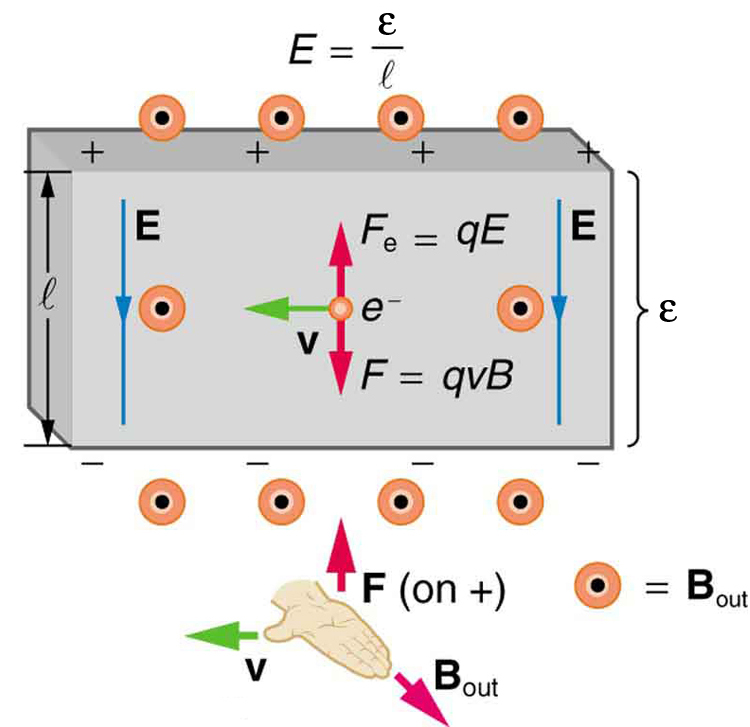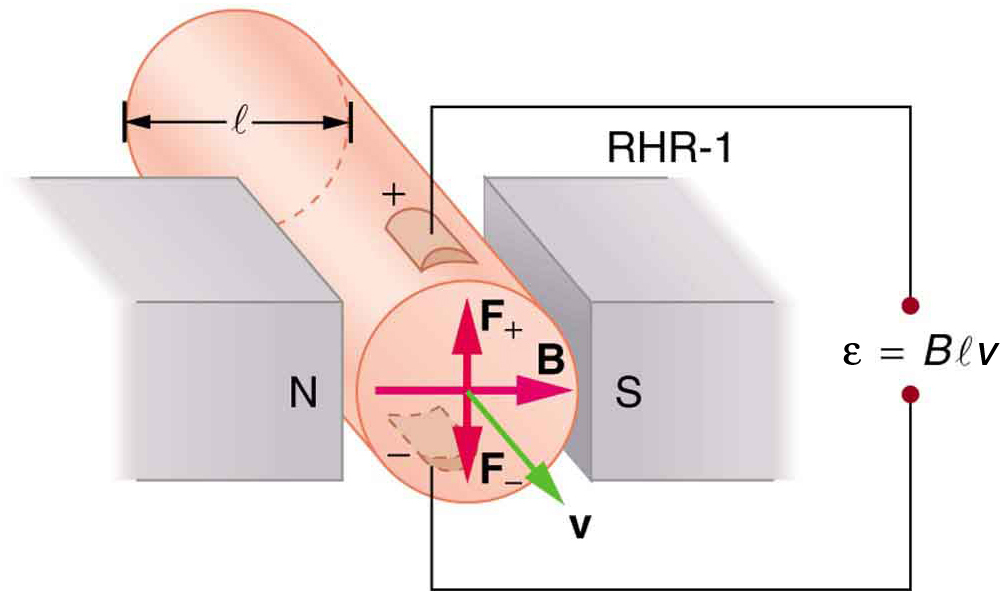# 22.6 The hall effect  (Page 2/4)

 Page 2 / 4
$\frac{\epsilon }{l}=\text{vB}\text{.}$

Solving this for the Hall emf yields

$\epsilon =\text{Blv}\phantom{\rule{0.25em}{0ex}}\left(B,\phantom{\rule{0.25em}{0ex}}v,\phantom{\rule{0.25em}{0ex}}\text{and}\phantom{\rule{0.25em}{0ex}}l,\phantom{\rule{0.25em}{0ex}}\text{mutually perpendicular}\right),$

where $\epsilon$ is the Hall effect voltage across a conductor of width $l$ through which charges move at a speed $v$ .The Hall emf ε size 12{ε} {} produces an electric force that balances the magnetic force on the moving charges. The magnetic force produces charge separation, which builds up until it is balanced by the electric force, an equilibrium that is quickly reached.

One of the most common uses of the Hall effect is in the measurement of magnetic field strength $B$ . Such devices, called Hall probes , can be made very small, allowing fine position mapping. Hall probes can also be made very accurate, usually accomplished by careful calibration. Another application of the Hall effect is to measure fluid flow in any fluid that has free charges (most do). (See [link] .) A magnetic field applied perpendicular to the flow direction produces a Hall emf $\epsilon$ as shown. Note that the sign of $\epsilon$ depends not on the sign of the charges, but only on the directions of $B$ and $v$ . The magnitude of the Hall emf is $\epsilon =\text{Blv}$ , where $l$ is the pipe diameter, so that the average velocity $v$ can be determined from $\epsilon$ providing the other factors are known.The Hall effect can be used to measure fluid flow in any fluid having free charges, such as blood. The Hall emf ε size 12{ε} {} is measured across the tube perpendicular to the applied magnetic field and is proportional to the average velocity v size 12{v} {} .

## Calculating the hall emf: hall effect for blood flow

A Hall effect flow probe is placed on an artery, applying a 0.100-T magnetic field across it, in a setup similar to that in [link] . What is the Hall emf, given the vessel’s inside diameter is 4.00 mm and the average blood velocity is 20.0 cm/s?

Strategy

Because $B$ , $v$ , and $l$ are mutually perpendicular, the equation $\epsilon =\text{Blv}$ can be used to find $\epsilon$ .

Solution

Entering the given values for $B$ , $v$ , and $l$ gives

$\begin{array}{lll}\epsilon & =& \text{Blv}=\left(\text{0.100 T}\right)\left(4\text{.}\text{00}×{\text{10}}^{-3}\phantom{\rule{0.25em}{0ex}}m\right)\left(0\text{.200 m/s}\right)\\ & =& \text{80.0 μV}\end{array}$

Discussion

This is the average voltage output. Instantaneous voltage varies with pulsating blood flow. The voltage is small in this type of measurement. $\epsilon$ is particularly difficult to measure, because there are voltages associated with heart action (ECG voltages) that are on the order of millivolts. In practice, this difficulty is overcome by applying an AC magnetic field, so that the Hall emf is AC with the same frequency. An amplifier can be very selective in picking out only the appropriate frequency, eliminating signals and noise at other frequencies.

## Section summary

• The Hall effect is the creation of voltage $\epsilon$ , known as the Hall emf, across a current-carrying conductor by a magnetic field.
• The Hall emf is given by
$\epsilon =\text{Blv}\phantom{\rule{0.25em}{0ex}}\left(B,\phantom{\rule{0.25em}{0ex}}v,\phantom{\rule{0.25em}{0ex}}\text{and}\phantom{\rule{0.25em}{0ex}}l,\phantom{\rule{0.25em}{0ex}}\text{mutually perpendicular}\right)$
for a conductor of width $l$ through which charges move at a speed $v$ .

## Conceptual questions

Discuss how the Hall effect could be used to obtain information on free charge density in a conductor. (Hint: Consider how drift velocity and current are related.)

## Problems&Exercises

A large water main is 2.50 m in diameter and the average water velocity is 6.00 m/s. Find the Hall voltage produced if the pipe runs perpendicular to the Earth’s $5\text{.}\text{00}×{\text{10}}^{-5}\text{-T}$ field.

$7\text{.}\text{50}×{\text{10}}^{-4}\phantom{\rule{0.25em}{0ex}}V$

What Hall voltage is produced by a 0.200-T field applied across a 2.60-cm-diameter aorta when blood velocity is 60.0 cm/s?

(a) What is the speed of a supersonic aircraft with a 17.0-m wingspan, if it experiences a 1.60-V Hall voltage between its wing tips when in level flight over the north magnetic pole, where the Earth’s field strength is $8\text{.}\text{00}×{\text{10}}^{-5}\phantom{\rule{0.25em}{0ex}}\text{T?}$ (b) Explain why very little current flows as a result of this Hall voltage.

(a) 1.18 × 10 3 m/s

(b) Once established, the Hall emf pushes charges one direction and the magnetic force acts in the opposite direction resulting in no net force on the charges. Therefore, no current flows in the direction of the Hall emf. This is the same as in a current-carrying conductor—current does not flow in the direction of the Hall emf.

A nonmechanical water meter could utilize the Hall effect by applying a magnetic field across a metal pipe and measuring the Hall voltage produced. What is the average fluid velocity in a 3.00-cm-diameter pipe, if a 0.500-T field across it creates a 60.0-mV Hall voltage?

Calculate the Hall voltage induced on a patient’s heart while being scanned by an MRI unit. Approximate the conducting path on the heart wall by a wire 7.50 cm long that moves at 10.0 cm/s perpendicular to a 1.50-T magnetic field.

11.3 mV

A Hall probe calibrated to read $1\text{.}\text{00 μV}$ when placed in a 2.00-T field is placed in a 0.150-T field. What is its output voltage?

Using information in [link] , what would the Hall voltage be if a 2.00-T field is applied across a 10-gauge copper wire (2.588 mm in diameter) carrying a 20.0-A current?

$1.\text{16 μV}$

Show that the Hall voltage across wires made of the same material, carrying identical currents, and subjected to the same magnetic field is inversely proportional to their diameters. (Hint: Consider how drift velocity depends on wire diameter.)

A patient with a pacemaker is mistakenly being scanned for an MRI image. A 10.0-cm-long section of pacemaker wire moves at a speed of 10.0 cm/s perpendicular to the MRI unit’s magnetic field and a 20.0-mV Hall voltage is induced. What is the magnetic field strength?

2.00 T

what is thermodynamics
thermodynamic is a branch of physics that teaches on the relationship about heat and anyother form of energy
Emmanuel
if l cary box and stop is ther any work
no that because u have moved no distance. for work to be performed a force needs to be applied and a distance needs to be moved
Emmanuel
Different between fundamental unit and derived unit
fundamental unit are independent quantities that do not depend on any other unit while derived unit are quantities that depend on two or more units for it definition
Emmanuel
what is nuclear fission
hello
Shawty
are you there
Shawty
Shawty
what is a vector
vectors are quantities that have numerical value or magnitude and direction.
what is regelation
vector is any quantity that has magnitude and direction
Emmanuel
Physics is a physical science that deals with the study of matter in relation to energy
Hi
Jimoh
hello
Salaudeen
hello
Yes
Maxamuud
hi everyone
what is physics
physics is a physical science that deals with the study of matter in relation to energy
Osayuwa
a15kg powerexerted by the foresafter 3second
what is displacement
movement in a direction
Jason
hello
Hosea
Hey
Smart
haider
Explain why magnetic damping might not be effective on an object made of several thin conducting layers separated by insulation? can someone please explain this i need it for my final exam
Hi
saeid
hi
Yimam
Hi
Jimoh
An object made of several thin conducting layers separated by insulation may not be affected by magnetic damping because the eddy current produced in each layer due to induction will be very small and the opposing magnetic flux produced by the eddy currents will be very small
What is thê principle behind movement of thê taps control
while
Hosea
what is atomic mass
this is the mass of an atom of an element in ratio with the mass of carbon-atom
Chukwuka
show me how to get the accuracies of the values of the resistors for the two circuits i.e for series and parallel sides
Explain why it is difficult to have an ideal machine in real life situations.
tell me
Promise
what's the s . i unit for couple?
Promise
its s.i unit is Nm
Covenant
Force×perpendicular distance N×m=Nm
Oluwakayode
İt iş diffucult to have idêal machine because of FRİCTİON definitely reduce thê efficiency
Oluwakayode
It is difficult to have an ideal machine in real life situation because in ideal machines all the input energy should be converted to output energy . But , some part of energy is always lost in overcoming friction and input energy is always greater than output energy . Hence , no machine is ideal.
if the classica theory of specific heat is valid,what would be the thermal energy of one kmol of copper at the debye temperature (for copper is 340k)

#### Get Jobilize Job Search Mobile App in your pocket Now!ByBy Angela JanuaryBy Ellie BanfieldBy OpenStaxBy OpenStaxBy Mahee BooByBy Robert MurphyBy Brooke DelaneyBy OpenStaxBy OpenStax